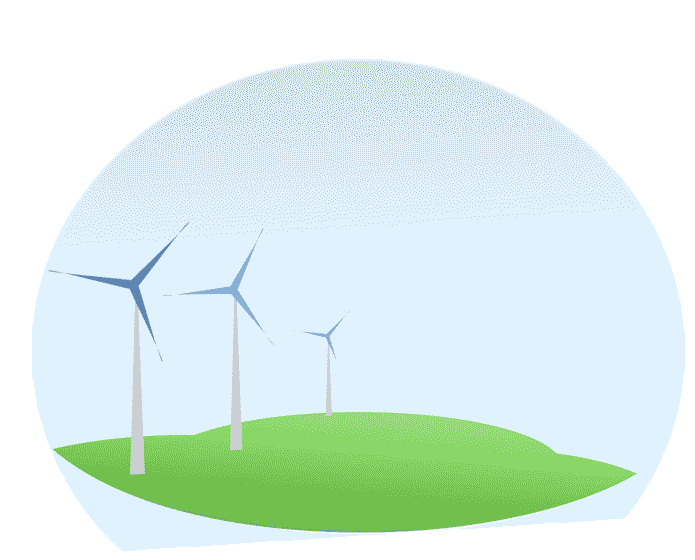•••Posted by Admin / in Science Articles

### Potential Energy

Potential energy is also known as gravitational energy. An object that is elevated above the ground has potential energy because if it is dropped it will start to move down because gravity pulls it to the ground. An object that is able to roll or slide down also has potential energy. A good example of potential energy is a snow skier. The skier is able to generate speed and travel a distance because of the starting height on top of a hill or mountain. The amount of potential energy an elevated object has varies based on the mass of the object and its height it can drop or roll down the hill. In this case, the skier is sliding down the mountain. Once the skier reaches the point where the grade is flat, he will no longer have potential energy and will stop accelerating and begin to slow down.Does a car traveling down a hill have potential energy?

PEgravitational = m x g x h

m = mass (weight in English units)

g = gravity constant (32.2 ft/s2)

h = height of the mass

### Kinetic Energy

Kinetic energy is the energy possible from a moving or rotating object. This energy principle would apply to accelerating the object or to bringing the object to a stop. Using the kinetic energy concept, the amount of energy necessary to make a 100 pound object move 20 mph is solved using the following formula:

Ekinetic = (mv)2/2g = ((100lb)x(20mph))2/2*32.2 ft/sec2) = 621.12

m = mass

v = velocity

g = gravity constant (32.2 ft/s2)

### Spring Energy

Spring Energy is energy stored in exactly what you may think, a spring! A spring can be used to store energy since they are capable of doing work. In an ideal spring, the amount of spring energy equals the amount of work required to compress or pull apart the spring. The spring energy is not dependent on the mass of the spring, it is actually dependent on the spring constant (stiffness), k.

Espring = ½(k(x)2

x = displacement

### Internal Energy

Internal energy typically involves heat transfer characteristics of a mass, also known as thermodynamics. The total internal energy of a mass (U) increases when its temperature increases. If there is no other work done to the mass or by the mass, the change in internal energy is equal to the heat flow (Q) into the mass.

U2 - U1 = Q

### Pressure Energy

Pressure energy is energy stored in the form of pressure. This pressure is typically gas, however, in some systems such as hydraulic systems, pressure energy can also involve liquids. An example of pressure energy is when a balloon is inflated. In this example, mechanical energy is stored as pressure energy.

In all these cases, once the energy is used up by a mass it will not get the energy back without the influence of additional energy.

MORE SCIENCE PLACES TO VISIT